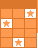Challenge Board: Score points by answering questions correctly.# Multiplication Mixed Bag

See if you can solve these varied multiplication problems and word problems. Use scrap paper if you need to. When writing the equations for the word problem answers, leave a space between each number and symbol (ie: 2 X 2 = 4 or 12 X 1 = 12. Notice that I did not put a space between the 1 and 2 in 12.). Also, make sure that the equation you write for the word problems uses the information from the problem, since there may be more than one equation (using different factors) that could give you the same product. Have fun!This activity was created by a Quia Web subscriber. Learn more about QuiaCreate your own activities